Next: Details of the Rating Up: The USCF Rating System Previous: The USCF Rating System

# The Rating Algorithm

Before an event, a player is either unrated, or has a rating based on having played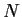games. A player's rating is termed provisional'' if it is based on 25 or fewer games, and is established'' otherwise. Assume the player competes in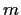games during the event. Post-event ratings are computed in a sequence of five steps:

• The first step sets temporary initial ratings for unrated players.
• The second step calculates an effective'' number of games played by each player.
• The third step calculates temporary estimates of ratings for unrated players only to be used when rating their opponents on the subsequent step.
• The fourth step then calculates intermediate ratings for all players.
• The fifth step uses these intermediate ratings from the previous step as estimates of opponents' strengths to calculate final post-event ratings.
The calculations are carried out in the following manner:

Step 1:
Set initial ratings for unrated players.

Initial rating estimates are set for all unrated players in an event. The purpose of setting initial rating estimates for unrated players is (1) to be able to incorporate information about a game result against an unrated player, and (2) to choose among equally plausible ratings during a rating calculation for an unrated player (see the details of the special'' rating formulas in Section 2.2.

An initial rating for an unrated player is determined in the following order of precedence.

• If an unrated player has a FIDE rating, use a converted rating according to the following formula: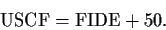If the FIDE rating is over 2150, then this converted rating is treated as based on having played 10 games (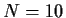). If the FIDE rating is 2150 or less, then this converted rating is treated as based on having played 5 games (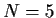).
• If an unrated player has a CFC rating over 1500, use a converted rating according to the following formula: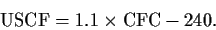This converted rating is treated as based on having played 5 games ().

If an unrated player has a CFC rating of 1500 or less, use a converted rating according to the following formula: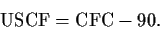This converted rating is treated as based on having played 0 games (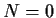).
• If the event is a regular event and the player has a QC rating (and does not have a standard rating) based on at least four games, use the QC rating as the imputed standard rating. The rating is assumed to be based on 0 games ().

Conversely, if the event is a QC event and the player only has a standard rating based on at least four games, use the standard rating as the imputed rating. The rating is assumed to be based on the lesser of 10 and the number of games upon which the standard rating is based (or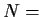number of prior QC games, whichever is smaller).

• Otherwise, impute an age-based rating according to the following procedure. Define a player's age (in years) to be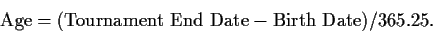The formula for an initial rating based on age is given by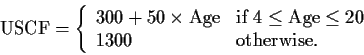The rating is assumed to be based on 0 games (). As a practical concern, if Age'' is calculated to be less than 4 years old, then it is assumed that a miscoding of the player's birthday occurred, so that such a player is treated as a 20-year old in the Age-based calculation.
• If no international rating or birth information is supplied, and if the player does not have a non-correspondence USCF rating, impute a rating of 750. This rating is assumed to be based on 0 games ().

Step 2:
Calculate the effective'' number of games played by each player.

This number, which is typically less than the actual number of games played, reflects the uncertainty in one's rating, and is substantially smaller especially when the player's rating is low. This value is used in the special'' and standard'' rating calculations. See Section 2.1 for the details of the computation.

Step 3:
Calculate a first rating estimate for each unrated player. For most unrated players, this involves using the special'' rating formula (see Section 2.2). Exceptions include unrated players with high FIDE ratings, and unrated quick chess players with standard ratings based on 9 or 10 games. Let the prior'' rating,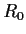, and the number of games,, be the values assigned from Step 1. However, for only this step in the computation, set the number of effective games to 1 for each unrated player having a Step 1 rating based ongames.
• If an opponent of the unrated player has a pre-event rating, use this rating in the special'' rating formula.
• If an opponent of the unrated player is also unrated, then use the initial rating imputed from Step 1.
If the resulting rating from Step 3 for the unrated player is less than 100, then change the rating to 100.

Step 4:
For every player, calculate an intermediate rating with the appropriate rating formula.
• If a player is unrated, use the special'' rating formula with initial prior'' rating,, from Step 1. The effective number of games for unrated players is the value determined from Step 2.
• If a player has played 8 or fewer games (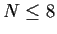), or if a player's game outcomes in all previous events have been either all wins or all losses, then use the special'' rating formula, with prior'' rating,, equal to the player's pre-event rating.
• If a player has completed more than 8 games (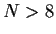) and has not had either all wins or all losses, use the standard'' rating formula (see Section 2.3).
In the calculations, use the opponents' pre-event ratings in the computation (for players with pre-event ratings), or the results of the Step 3 ratings for unrated opponents.

If the resulting rating from Step 4 is less than 100, then change the rating to 100.

Step 5:
Repeat the calculations from Step 4 for every player, again using a player's pre-event rating (or the results of Step 1 for unrated players) to perform the calculation, but using the results of Step 4 for the opponents' ratings. If the resulting rating from Step 5 is less than 100, then change the rating to 100.

These five steps result in the new set of post-event ratings for all players.Next: Details of the Rating Up: The USCF Rating System Previous: The USCF Rating System
Mark Glickman
2004-09-22